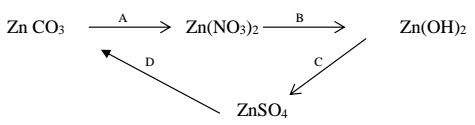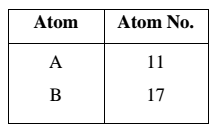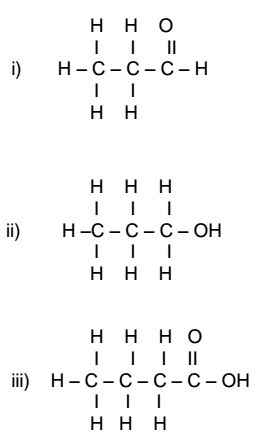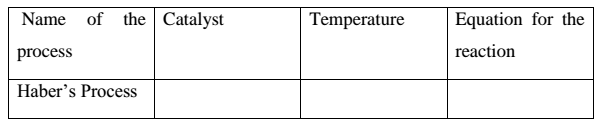# ICSE Class 10 Chemistry 2019 Sample Paper

Class 10 Chemistry

Chemistry is the Science Paper - 2. The duration of the exam is two hours. Section I is compulsory. You have to attempt any four questions from Section II.

### Section (40 Marks)

Question 1

(a) Choose the correct answer from the options given below: 

(i) A strong electrolyte from the following is :
A. Acetic acid
B. Oxalic acid
C. Ammonium hydroxide
D. Sodium hydroxide

(ii) Electron affinity is maximum in:
A. Alkali metals
B. Alkaline earth metals
C. Halogens
D. Inert gases

(iii) The main components of brass are :
A. Copper and Zinc
C. Copper and tin
D. Copper and iron

(iv) The drying agent used to dry NH3 is:
A. P2O5
B. conc. H2SO4
C. CaCl2
D. CaO

(v) The general formula of alkynes is:
A. CnH2n–2
B. CnH2n+2
C. CnH2n
D. CnH2n+2O

(b) Write balanced chemical equations for each of the following: 

(i) Catalytic oxidation of ammonia
(ii) Action of concentrated nitric acid on Sulphur.
(iii) Action of concentrated sodium hydroxide on Zinc oxide
(iv) Reaction between acetic acid with ethanol in the presence of concentrated sulphuric acid.
(v) Action of dilute hydrochloric acid on iron.

(c) State any one observation for each of the following: 

(i) Dilute Hydrochloric acid is added to Silver Nitrate solution.
(ii) Concentrated Nitric acid is added to Copper turnings.
(iii) Mixture of Ammonium Chloride and Sodium Hydroxide is heated.
(iv) Ammonium hydroxide solution is added in excess to copper sulphate solution.
(v) NaOH solution is added to calcium nitrate solution.

(d) Rewrite the following by inserting appropriate word / words: 

(i) Magnesium Nitride reacts with water to liberate Ammonia.
(iii) Starch iodide paper turns blue black in the presence of Chlorine.
(iv) Hydrogen chloride molecule contains a covalent bond.
(v) Acid salts are formed by replacement of the ionisable hydrogen ions of the acid by a metallic ion or ammonium ion.

(e) 

(i) Given: 2C2H6 + 7O2 → 4CO2 + 6H2O
2000 cc of O2 was burnt with 400 cc of ethane. Calculate the volume of CO2 formed and unused O2
(ii) Find the number of moles and molecules present in 7.1 g of Cl2. (At. Wt. Cl = 35.5)
(iii) Calculate the vapour density of ethene [C =12 , H = 1]

(f) Identify the terms: 

(i) The energy required to remove an electron from valance shell of a neutral isolated gaseous atom.
(ii) The method for the concentration of sulphide ores.
(iii) The property by which carbon bonds with itself to form a long chain.
(iv) A bond formed by a shared pair of electrons with both electrons coming from the same atom.
(v) A substance that conducts electricity in molten or aqueous state.

(g) Arrange the following as per the instruction given in the brackets. 
(i) Li, F, N [increasing order of electronegativity]
(ii) Na, Al , Cl [increasing order of Ionization potential ]
(iii) O2, N2, Cl2 [increasing order of number of covalent bonds]
(iv) Zn2+, Na+, Cu+2 [order of preference of discharge at the cathode]
(v) Br , F , Cl [Decreasing order of atomic radius]

(h) 

(i) Draw the structural formula for each of the following:
1. But-1-ene
2. Propanoic acid
3. Ethanol
(ii) Draw the structural isomers of C4H10

### Section (40 Marks)

Question 2

(a) Write balanced equations for following conversions:(b) Show the formation of H3O+ using the electron dot diagram. State the types of bonds present in it. 

(c) Distinguish between the following pairs of compounds using the test given within the brackets. 

(i) Calcium sulphite and calcium carbonate (using dil. HCl)
(ii) Calcium nitrate and potassium nitrate (using a flame test)
(iii) Lead nitrate solution and Zinc nitrate solution (using an alkali)

Question 3

(a) Study the table and answer the following questions:(i) Compare the positions of A and B in the Periodic Table.
(ii) Which is more metallic?
(iii) Write equations for the formation of ions of A and B.
(iv) What type of bond is formed between A and B? Mention its physical state and solubility in water.

(b) Identify the gas evolved in each of the following cases: 

(i) A colorless gas liberated on decomposition of nitric acid.
(ii) Water is added to calcium carbide
(iii) Dilute hydrochloric acid is added to Zinc sulphide.
(iv) Dilute nitric acid is added to copper.

Question 4

(a) Write a balanced equation for the following: 

(i) C2H5Br + alcoholic KOH →
(ii) CH3CH2COONa + soda lime →
(iii) C2H4 + Br2 →
(iv) C2H5OH + Na →

(b) State how the following conversions can be carried out. 

(i) Ethyl chloride to ethyl alcohol
(ii) Ethyl alcohol to ethene.
(iii) Ethyl bromide to ethane.

(c) Give the correct IUPAC name for each of the compounds whose structural formulae are given below.Question 5

(a) (i) Name the chief ore of Aluminum and the process of concentration of the ore.
(ii) Write balanced equations for the conversion of the above ore of aluminum to pure alumina.
(iii) Name one alloy of Aluminum. 

(b) A compound gave a following data: 
C = 57.82%, O = 38.58% and the rest hydrogen. Its relative molecular mass is 166.
Find its empirical formula and molecular formula. [C =12, O = 16, H = 1]

Question 6

(a) (i) Copy and complete the following table:(ii) How is Ammonia separated from unreacted Nitrogen and Hydrogen?

(b) Give appropriate scientific reasons for each of the following statements. 

(i) Electrolysis of molten lead bromide is considered to be a redox reaction.
(ii) Although copper is a good conductor of electricity it is a non-electrolyte.
(iii) Electrical conductivity of acetic acid is less in comparison to that of dil. Sulphuric acid.

(c) Mention the property of conc. H2SO4 exhibited in each of the following reactions with: 

(i) sugar
(ii) metallic chloride
(iii) non-metal such as carbon.

Question 7

(a) Answer the following questions pertaining to laboratory preparation of Hydrogen chloride: 

(i) Write an equation for the laboratory preparation of Hydrogen Chloride.
(ii) Name the drying agent used.
(iii) Name the method of collecting Hydrogen Chloride gas.
(iv) Give a test to identify the gas.

(b) Give reasons for each of the following: 

(i) Direct absorption of HCl gas in water is not preferred.
(ii) All glass apparatus is used in the laboratory preparation of HNO3.
(iii) NaCl has a high melting point.

(c) Give one point of difference between the following pairs of terms given: 

(i) Calcination and Roasting.
(ii) Polar and Non Polar covalent compounds.
(iii) Strong electrolyte and weak electrolyte.